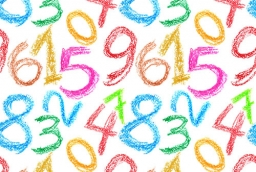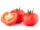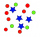# The number 4

Two-thirds of a number is negative six. Find the number.

x =  -9

### Step-by-step explanation:Did you find an error or inaccuracy? Feel free to write us. Thank you!Tips to related online calculators
Need help to calculate sum, simplify or multiply fractions? Try our fraction calculator.
Do you have a linear equation or system of equations and looking for its solution? Or do you have a quadratic equation?

## Related math problems and questions:

• Two thirdsFind two-thirds of the number equal to two-thirds of 99
• Expressions with variableThis is algebra. Let n represent an unknown number and write the following expressions: 1. 4 times the sum of 7 and the number x 2. 4 times 7 plus the number x 3. 7 less than the product of 4 and the number x 4. 7 times the quantity 4 more than the number
• Two thirdsTwo thirds of the unknown number is 8/7. What is 7/3 of this number?
• Unknown number4/5 of a number is 276. what is 2/3 of the same number?
• Multiplication negativesJosh says that (4)(-7)(-1) is equal to (4)(7). Evangelina says (4)(-7)(-1) is equal to (-28)(-1) . Who is correct? Explain your reasoning.
• Equivalent equationWhich equation, when solved, results in a different value of x than the other three? A. - (7)/(8) x - (3)/(4) = 20 Negative 7/8 x minus three-fourths = 20 B. (3)/(4) + (7)/(8) x= -20 Three-fourths + StartFraction 7 over 8 En
• The ketchupIf 3 1/4 of tomatoes are needed to make 1 bottle of ketchup. Find the number of tomatoes required to make 4 1/5 bottles
• Algebra problemThis is algebra. Let n represent an unknown number. 1. Eight more than the number n 2. Three times the number n 3. The product of the number n and eight 4. Three less than the number n 5. Three decreased by the number n
• Numbers divisionWith what number should be divided mixed number 2 3/4 to get 11/12?
• Equation with fractionsWhat is another way to write the equation 7/8 x + three-fourths = negative 6?
• Three digits number 2Find the number of all three-digit positive integers that can be put together from digits 1,2,3,4 and which are subject to the same time has the following conditions: on one positions is one of the numbers 1,3,4, on the place of hundreds 4 or 2.
• The ratio 4The ratio of two number is 5:4 if 40% of the first number is 12, what will be 50% of the second number?
• Fraction of a NumberIf 1/2 of 1/3 of 1/4 of 1/5 of a number is 2.5. what is the number?
• CompareCompare fractions (34)/(3) and (12)/(4). Which fraction of the lower?
• Negative in equation2x + 3 + 7x = – 24, what is the value of x?
• The product 2The product of two functions is 10. If one of them is 2 1/3, find the other one.
• FractionFind for what x fraction (-4x -6)/(x) equals: